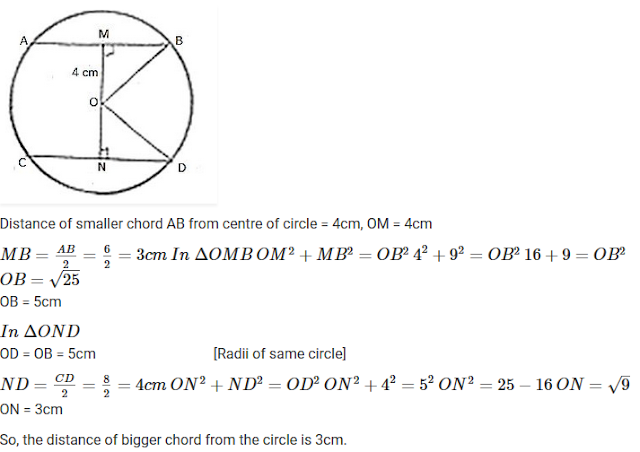#### Chapter 16 Circles R.D. Sharma Solutions for Class 9th Exercise 16.2

Exercise 16.2

1. The radius of a circle is 8 cm and the length of one of its chords is 12 cm. Find the distance of the chord from the centre.

Solution2. Find the length of a chord which is at a distance of 5 cm from the centre of a circle of radius 10 cm.

Solution2.  Find the length of a chord which is at a distance of 4 cm from the centre of the circle of radius 6 cm.

Solution3. Find the length of a chord which is at a distance of 4 cm from the centre of the circle of radius 6 cm.

Solution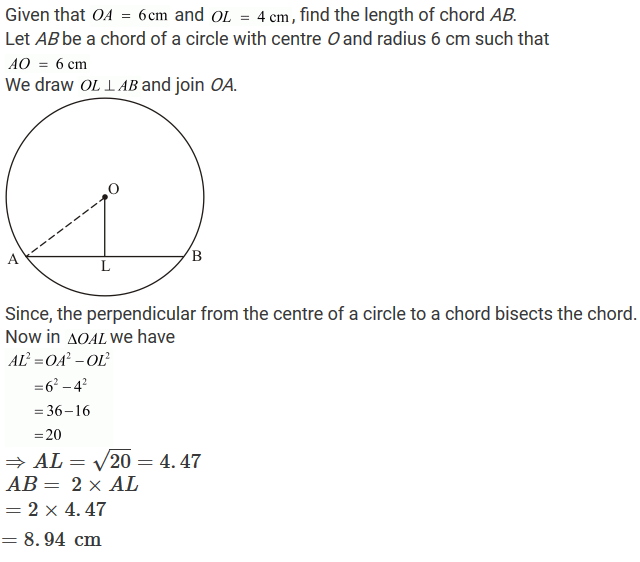4. Two chords AB, CD of lengths 5 cm, 11 cm respectively of a circle are parallel, If the distance between AB and CD is 3 cm, find the radius of the circle.

Solution5. Give a method to find the centre of a given circle.

Solution
Let AB and C are three distinct points on a circle.
Now join AB and BC and draw their perpendicular bisectors.
The point of intersection of the perpendicular bisectors is the centre of given circle.
Hence O is the centre of circle C(0,r).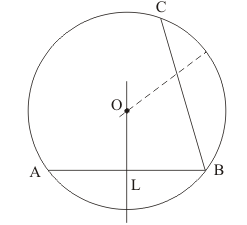6. Prove that the line joining the mid-point of a chord to the centre of the circle passes through the mid-point of the corresponding minor arc.

Solution7. Prove that a diameter of a circle which bisects a chord of the circle also bisects the angle subtended by the chord at the centre of the circle.

Solution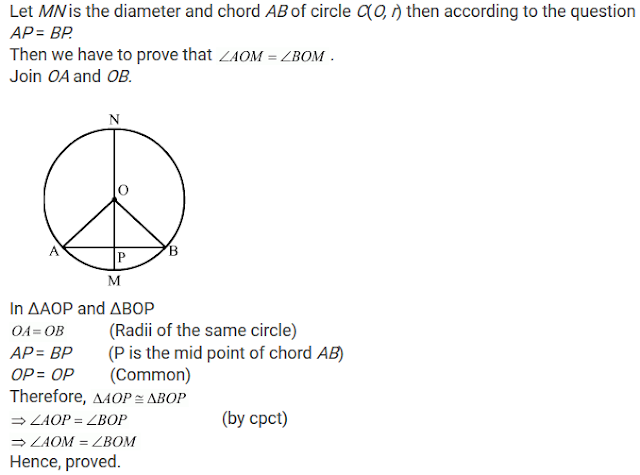8. Prove that two different circles cannot intersect each other at more than two points .

Solution

We have to prove that two different circles cannot intersect each other at more than two points.
Let the two circles intersect in three points A, B and C.
Then as we know that these three points A, B and C are non-collinear. So, a unique circle passes through these three points.
This is a contradiction to the fact that two given circles are passing through A, B, C.
Hence, two circles cannot intersect each other at more than two points.
Hence, proved.

9. A line segment AB is of length 5cm. Draw a circle of radius 4 cm passing through A and B. Can you draw a circle of radius 2 cm passing through A and B? Give reason in support of your answer.

Solution

Given that, a line AB = 5 cm, one circle having radius r1 = 4 cm which is passing through point A and Band other circle of radius r2 = 2 cm.
As we know that the largest chord of any circle is equal to the diameter of that circle.
So, 2×r2<AB
There is no possibility to draw a circle whose diameter is smaller than the length of the chord.

10. An equilateral triangle of side 9 cm is inscribed in a circle. Find the radius of the circle.

Solution11. Given an arc of a circle, complete the circle.

Solution

Let PQ be an arc of the circle.
In order to complete the circle. First of all we have to find out its centre and radius.
Now take a point R on the arc PQ and join PR and QR.
Draw the perpendicular bisectors of PR and QR respectively.
Let these perpendicular bisectors intersect at point O.
Then OP = OQ, draw a circle with centre O and radius OP = OQ to get the required circle12. Draw different pairs of circles. How many points does each pair have in common? What is the maximum number of common points?

Solution13. Suppose you are given a circle. Give a construction to find its centre.

Solution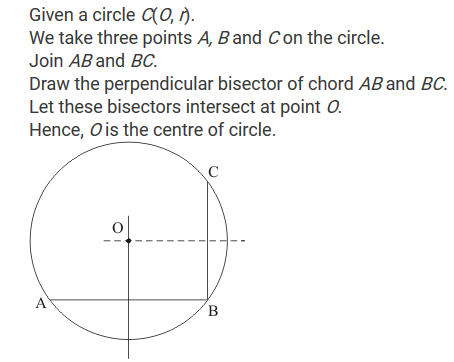14. Two chords AB and CD of lengths 5 cm and 11 cm respectively of a circle are parallel to each other and are opposite side of its centre. If the distance between AB and CD is 6 cm, find the radius of the circle.

Solution15. The lengths of two parallel chords of a circle are 6 cm and 8 cm. If the smaller chord is at a distance of 4 cm from the centre, what is the distance of the other chord from the centre?

Solution